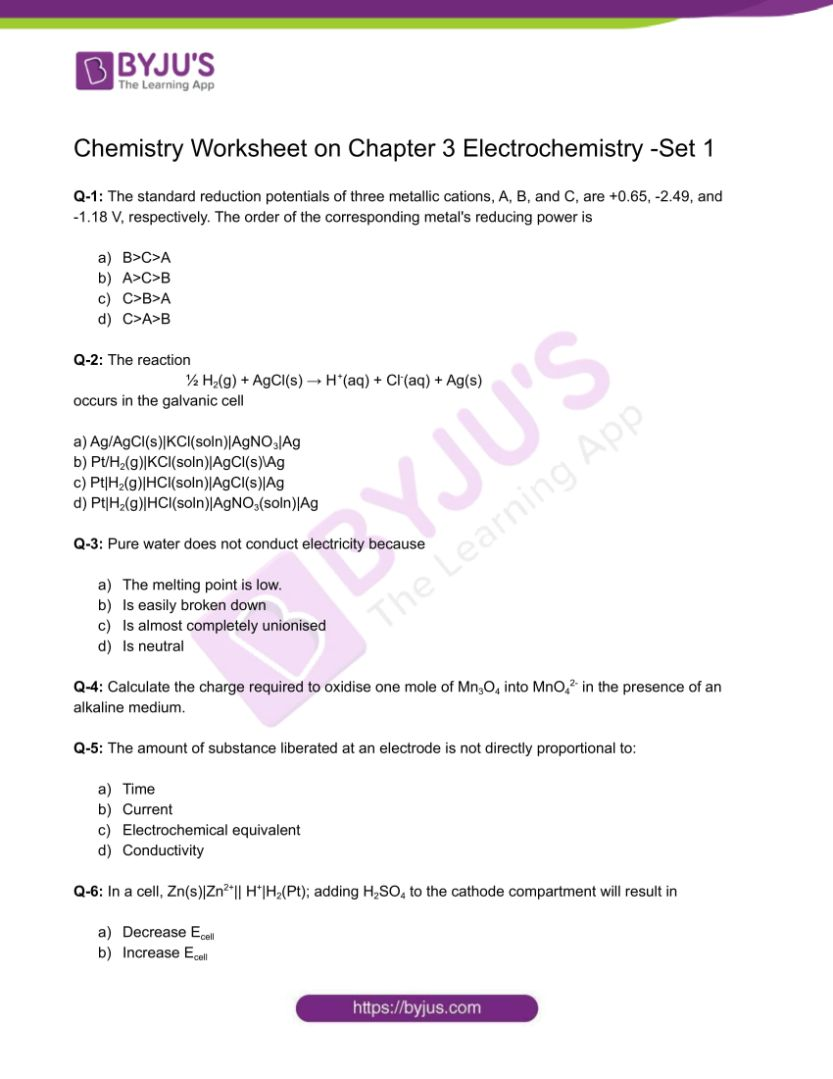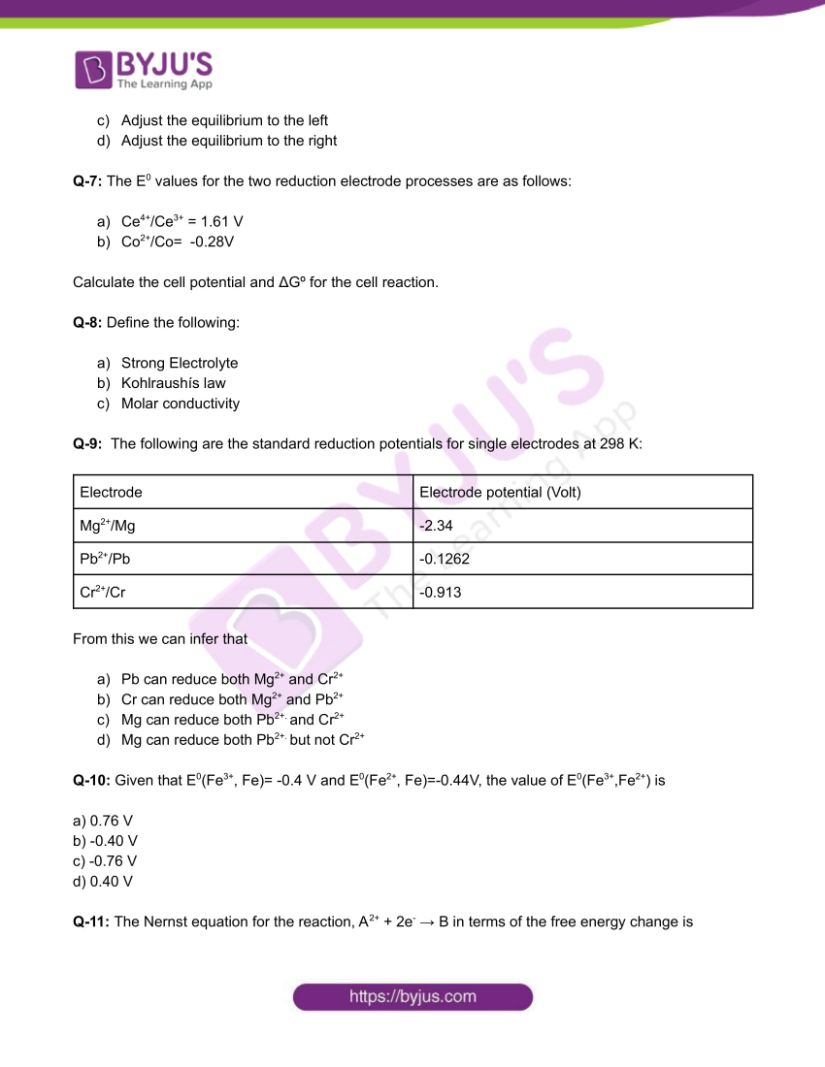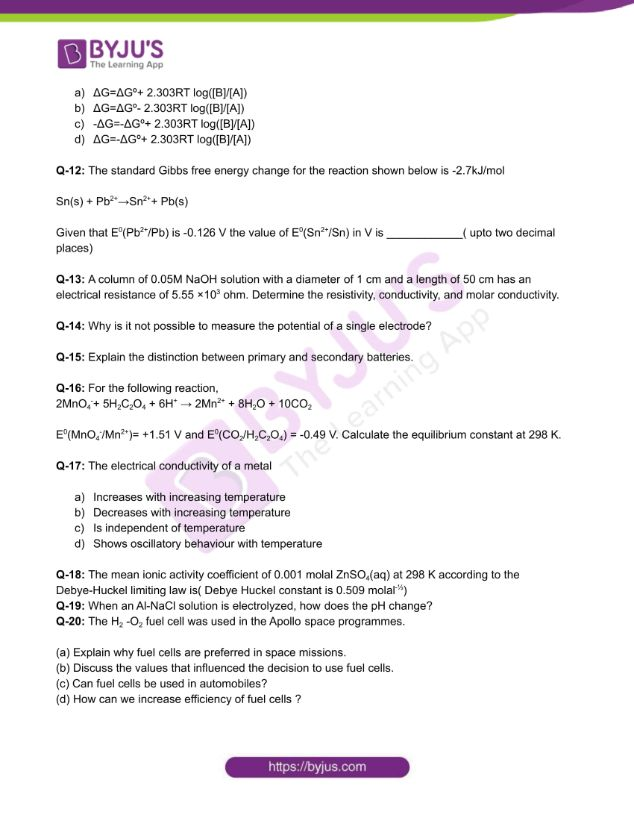Checkout JEE MAINS 2022 Question Paper Analysis : Checkout JEE MAINS 2022 Question Paper Analysis :

# Chemistry Worksheets Class 12 on Chapter 3 Electrochemistry with Answers - Set 1

Electrochemistry is the study of the generation of electricity from the energy released during spontaneous chemical reactions, as well as the application of electrical energy to cause non-spontaneous chemical reactions transformations. The subject is significant on both counts for both theoretical and practical reasons. A large variety of metals, sodium hydroxide, chlorine and Fluorine and a variety of other chemicals are created by electrochemical techniques.

## 👇## CBSE Class 12 Chemistry Chapter 3 Electrochemistry Worksheet – Set 1

Q-1: The standard reduction potentials of three metallic cations, A, B, and C, are +0.65, -2.49, and -1.18 V, respectively. The order of the corresponding metal’s reducing power is

1. B>C>A
2. A>C>B
3. C>B>A
4. C>A>B

Q-2: The reaction

½ H2(g) + AgCl(s) → H+(aq) + Cl(aq) + Ag(s)

occurs in the galvanic cell

a) Ag/AgCl(s)|KCl(soln)|AgNO3|Ag

b) Pt/H2(g)|KCl(soln)|AgCl(s)\Ag

c) Pt|H2(g)|HCl(soln)|AgCl(s)|Ag

d) Pt|H2(g)|HCl(soln)|AgNO3(soln)|Ag

Q-3: Pure water does not conduct electricity because

1. The melting point is low.
2. Is easily broken down
3. Is almost completely unionised
4. Is neutral

Q-4: Calculate the charge required to oxidise one mole of Mn3O4 into MnO42- in the presence of an alkaline medium.

Q-5: The amount of substance liberated at an electrode is not directly proportional to:

1. Time
2. Current
3. Electrochemical equivalent
4. Conductivity

Q-6: In a cell, Zn(s)|Zn2+|| H+|H2(Pt); adding H2SO4 to the cathode compartment will result in

1. Decrease Ecell
2. Increase Ecell
3. Adjust the equilibrium to the left
4. Adjust the equilibrium to the right

Q-7: The E0 values for the two reduction electrode processes are as follows:

1. Ce4+/Ce3+ = 1.61 V
2. Co2+/Co= -0.28V

Calculate the cell potential and ΔGº for the cell reaction.

Q-8: Define the following:

1. Strong Electrolyte
2. Kohlraushís law
3. Molar conductivity

Q-9: The following are the standard reduction potentials for single electrodes at 298 K:

Electrode Electrode potential (Volt)
Mg2+/Mg -2.34
Pb2+/Pb -0.1262
Cr2+/Cr -0.913

From this we can infer that

1. Pb can reduce both Mg2+ and Cr2+
2. Cr can reduce both Mg2+ and Pb2+
3. Mg can reduce both Pb2+. and Cr2+
4. Mg can reduce both Pb2+. but not Cr2+

Q-10: Given that E0(Fe3+, Fe)= -0.4 V and E0(Fe2+, Fe)=-0.44V, the value of E0(Fe3+,Fe2+) is

a) 0.76 V

b) -0.40 V

c) -0.76 V

d) 0.40 V

Q-11: The Nernst equation for the reaction, A2+ + 2e → B in terms of the free energy change is

1. ΔG=ΔGº+ 2.303RT log([B]/[A])
2. ΔG=ΔGº- 2.303RT log([B]/[A])
3. -ΔG=-ΔGº+ 2.303RT log([B]/[A])
4. ΔG=-ΔGº+ 2.303RT log([B]/[A])

Q-12: The standard Gibbs free energy change for the reaction shown below is -2.7kJ/mol

Sn(s) + Pb2+→Sn2++ Pb(s)

Given that E0(Pb2+/Pb) is -0.126 V the value of E0(Sn2+/Sn) in V is ____________( upto two decimal places)

Q-13: A column of 0.05M NaOH solution with a diameter of 1 cm and a length of 50 cm has an electrical resistance of 5.55 ×103 ohm. Determine the resistivity, conductivity, and molar conductivity.

Q-14: Why is it not possible to measure the potential of a single electrode?

Q-15: Explain the distinction between primary and secondary batteries.

Q-16: For the following reaction,

2MnO4+ 5H2C2O4 + 6H+ → 2Mn2+ + 8H2O + 10CO2

E0(MnO4/Mn2+)= +1.51 V and E0(CO2/H2C2O4) = -0.49 V. Calculate the equilibrium constant at 298 K.

Q-17: The electrical conductivity of a metal

1. Increases with increasing temperature
2. Decreases with increasing temperature
3. Is independent of temperature
4. Shows oscillatory behaviour with temperature

Q-18: The mean ionic activity coefficient of 0.001 molal ZnSO4(aq) at 298 K according to the Debye-Huckel limiting law is( Debye Huckel constant is 0.509 molal)

Q-19: When an Al-NaCl solution is electrolyzed, how does the pH change?

Q-20: The H2 -O2 fuel cell was used in the Apollo space programmes.

(a) Explain why fuel cells are preferred in space missions.

(b) Discuss the values that influenced the decision to use fuel cells.

(c) Can fuel cells be used in automobiles?

(d) How can we increase efficiency of fuel cells ?

Download the PDF to access answers to the Chemistry Worksheet for Class 12 Chemistry Chapter 3 Electrochemistry Set -1.